Pool coating

How many pieces of tiles 25 cm × 15 cm need to coat the bottom and side walls of the pool with bottom dimensions 30 m × 5 m, if the pool can fit up to 271500 liters of water?

Result

n =  7379

Solution:Leave us a comment of this math problem and its solution (i.e. if it is still somewhat unclear...):Be the first to comment!Next similar math problems:

1. Soccer team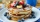Hilahs soccer team is trying to raise \$2414 to travel to a tournament in Florida, so they decided to host a pancake for the breakfast. How many people need to attend their breakfast in order to raise \$2414, if profit per one pancake is \$1.5?
2. Cage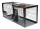How many m2 of mesh farmer use for fencing of a cuboid cage with dimensions 25m, 18m, and 2.5m?
3. AlmondsRudi has 4 cups of almonds. His trail mix recipe calls for 2/3 cup of almonds. How many batches of trail mix can he make?
4. DisjointHow many elements have union and intersection of two disjoint sets when the first have 1 and secodn 8 elements.
5. Passenger boatTwo-fifths of the passengers in the passenger boat were boys. 1/3 of them were girls and the rest were adult. If there were 60 passengers in the boat, how many more boys than adult were there?
6. Fire tankHow deep is the fire tank with the dimensions of the bottom 7m and 12m, when filled with 420 m3 of water?
7. CuboidCuboid has a surface of 516 cm2. Side a = 6 cm and b = 12 cm. How long is the side c =?
8. Prism 4 sidesFind the surface area and volume four-sided prism high 10cm if its base is a rectangle measuring 8 cm and 1.2dm
9. CustomerA customer purchase three (3) writing pads from a store. She receive k9.70 change from a k10.00 note . How much was it's writing pad cost?
10. Cuboid box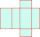Cuboid box have dimensions of 30 cm, 25 cm and 40 cm. Sketch its network and compute surface area of box.
11. Cuboid - VabFind the surface of the cuboid when its volume is 52.8 cubic centimeters, and the length of its two edges is 2 centimeters and 6 centimeters.
12. Cuboid surfaceDetermine surface area of cuboid if its volume is 52.8 cm cubic and length of the two edges are 2 cm and 6 cm.
13. Cuboid - simple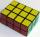Calculate the surface area and volume of a cuboid if a = 8 cm, b = 14 cm and c = 6 cm.
14. Surface of cuboidFind the surface of the cuboid if its volume is 52.8 cm3 and the length of its two edges is 2 cm and 6 cm.
15. Bulbs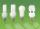In the box are 6 bulbs with power 75 W, 14 bulbs with power 40 W and 15 with 60 W. Calculate probability that a randomly selected bulb is:
16. Round it0.728 round to units, tenths, hundredths.
17. Cube surface areaWall of cube has content area 99 cm square. What is the surface of the cube?